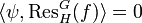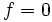Artin's induction theorem

This article states an induction theorem: a result relating the linear characters and linear representations of a group with the characters/representations induced from the linear characters/representations of subgroups
View a complete list of induction theorems
This fact is related to: linear representation theory
View other facts related to linear representation theoryView terms related to linear representation theory |

Statement

Let$G$ be a finite group and$X$ a family of subgroups of$G$. Then the following are equivalent:

1. The union of conjugates of elements of$X$ cover the whole of$G$
2. Every character of$G$ over$\mathbb{C}$ is a rational linear combination of characters induced from characters of members of$X$

Further, these equivalent conditions hold if$X$ is the collection of all cyclic subgroups of$G$.

Proof

This proof uses a tabular format for presentation. Provide feedback on tabular proof formats in a survey (opens in new window/tab) | Learn more about tabular proof formats|View all pages on facts with proofs in tabular format

Proof idea

With a little linear algebra, we can show that if a character of$G$ is a complex linear combination of characters induced from members of$X$, all the coefficients are in fact rational. Thus, the problem reduces to showing that the class functions induced from members of$X$ span the space of all class functions on$G$.

This proof follows by using Frobenius reciprocity, and the fact that the only class function on$G$ which restricts to the zero function on every member of$X$, is the zero function on the whole of$G$.

Proof details: (1) implies (2)

Given: A finite group$G$ with a family of subgroups$X$ such that the union of conjugates of members of$X$ is$G$.

To prove: Every character of$G$ is a rational linear combination of characters induced from characters of members of$X$.

Proof:

Step no. Assertion/construction Given data used Facts used Previous steps used Explanation
1 The$\mathbb{Q}$ (rational) vector space span of the irreducible characters contain all the characters. [SHOW MORE]
2 A subset of the$\mathbb{Q}$-span of characters with the property that its$\mathbb{C}$-span contains all class functions also has the property that its$\mathbb{Q}$-span contains all characters. Something about linear algebra; fields are linearly closed (1)
3 To show what we need to prove, it suffices to show that every class function is a$\mathbb{C}$-linear combination of characters induced from members of$X$. (1), (2) [SHOW MORE]
4 To show what we need to prove, it suffices to show that every class function is a$\mathbb{C}$-linear combination of class functions induced from members of$X$. (3) [SHOW MORE]
5 Let$V$ be the$\mathbb{C}$-span of all the class functions of$G$.$V$ is also the space of class functions of$G$. Let$W$ be the span of all class functions induced from characters of members of$X$. In other words:$\! W = \langle \operatorname{Ind}_H^G \psi \mid H \in X \rangle$.
6 Let$U = W^\perp$ be the orthogonal complement to$W$ in$V$ with respect to the inner product of class functions:$\! \langle \alpha,\beta \rangle = \frac{1}{|G|} \sum_{g \in G} \alpha(g) \overline{\beta(g)}$ (5)
7 Suppose$f \in U$. Then, for any$H \in X$ and any class function$\psi$ of$H$, we have:$\! \langle \operatorname{Ind}_H^G (\psi), f \rangle = \langle \psi, \operatorname{Res}_H^G(f) \rangle$. Fact (1) (Frobenius reciprocity)
8$\langle \operatorname{Ind}_H^G (\psi), f \rangle = 0$ (5), (6) [SHOW MORE]
9$\langle \psi, \operatorname{Res}_H^G(f) \rangle = 0$ (7), (8) [SHOW MORE]
10$\operatorname{Res}_H^G(f)$ is orthogonal to every class function of$H$. (9) [SHOW MORE]
11$\operatorname{Res}_H^G(f) = 0$ for every$H \in X$ The inner product is an inner product. (10) [SHOW MORE]
12$f(g) = 0$ for every element of$g$ in the union of conjugates of members of$X$. (6), (7):$f$ is a class function; (11) [SHOW MORE]
13$f$ is identically the zero function$G$ is the union of all conjugates of members of$X$ (12) [SHOW MORE]
14$W^\perp = 0$ (13) and (7) [SHOW MORE]
15$W = V$, i.e., every class function on$G$ is in the$\mathbb{C}$-span of those induced from class functions on members of$X$. (14), (5), and (6) [SHOW MORE]
16 We are done. (4) and (15) Follows directly.

Proof details: (2) implies (1)

The proof here is essentially the same; it uses Frobenius reciprocity to reason in the opposite direction.

Given: A finite group$G$ with a family of subgroups$X$ such that every character of$G$ is a rational linear combination of characters induced from$X$.

To prove:$G$ is the union of conjugates of members of$X$.

Proof: Since every character of$G$ is a rational linear combination of the characters induced from$X$, it is in particular true that the$\mathbb{C}$-span of class functions induced from class functions of$X$, is the whole space of class functions on$G$.

Taking the usual inner product of class functions:$\langle \alpha,\beta \rangle = \frac{1}{|G|} \sum_{g \in G} \alpha(g) \overline{\beta(g)}$.

Now, suppose$f$ is a class function of$G$ that takes the value$0$ on the union of conjugates of$H$ and is$1$ outside. Then we have that for every$H \in X$ and every class function$\psi$ of$H$:$\langle \psi, \operatorname{Res}_H^G f \rangle = 0$.

By Frobenius reciprocity, we get:$\langle \operatorname{Ind}_H^G (\psi), f \rangle = 0$.

In other words,$f$ is orthogonal to all the class functions induced from members of$H$. By assumption,$f$ is thus orthogonal to every class functino of$G$, forcing$f = 0$. By te way we defined$f$, we obtain that the union of conjugates of$H \in X$ must be the whole group$G$.

Proof details for the additional observation

The additional observation follows from fact (2): every group is a union of cyclic subgroups.